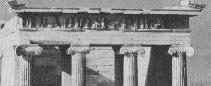# Frieze Patterns

From Encyclopædia Britannica:frieze, in classical architecture, the middle of the three main divisions of an entablature (q.v.; section resting on the capital), above the architrave and below the cornice.

In mathematics, frieze patterns have translational symmetry. Groups of isometries that keep a given straight line invariant and that include translations along the line, are called frieze groups with center at this line. Isometry is a linear transformation of the plane (or space) that preserve distances between points. Translation, rotation, reflection (in a point or a line) are all isometries. So are their compositions. Glide reflection is a simultaneous translation and reflection in the center line. In all, there are 7 frieze patterns. They are listed in the table below along with the corresponding kinds of symmetry.

 Frieze Patterns Type of Symmetry Point Center  Line Perpendicular  Line Glide  Reflection F1 LLLLLLLL F2 NNNNNN x F3 DDDDDDD x x F4 VVVVVVV x F5 HHHHHHH x x x x F6 ΛV ΛV ΛV x x x F7 ΓL ΓL ΓL xFrieze patterns found their way into mathematics through simple arithmetic. On a lattice, nodes are assigned integer values that obey certain rules. Variants of the rules apply to 4 numbers at the vertices of every small diamond (a,b,c,d). In the multiplicative case (x), the product of a and d is one more than the product of b and c: ad = bc + 1. In the additive case (+), the same is true of the sum: a+d = b+c+1. In a simpler case, 1 is omitted.

In the applet below, you can manipulated the numbers at the nodes of a lattice by just clicking on them. If you click a little to the right from a nodes center, the number will increase by 1. Clicking a little to the left decreases the number by 1. Start with several numbers on the left of the grid. Then press "Compute." The applet will propagate the numbers according to the selected rule.

You can clear the lattice by pressing either of the operation signs ("+" or "x") even the currently selected one. Selection of the modulus has only effect on the number of colors used. Maximum number of colors is 10. It's quite interesting to observe various number patterns by changing the basis of the arithmetic used for color selection.

In the multiplicative case, attempts to solve the equation ad = bc + 1 may lead to noninteger numbers. In which case I just drop the fractional part. Sometimes this may lead to zero nodes - a sure pattern killer.

### If you are reading this, your browser is not set to run Java applets. Try IE11 or Safari and declare the site https://www.cut-the-knot.org as trusted in the Java setup.Some of the mathematics related to these (arithmetic) frieze patterns is quite simple. Other is not. John Conway, currently of Princeton, found a way [The Book of Numbers, pp. 96-100] to associate multiplicative frieze patterns with such diverse objects as triangulations of convex polygons, binary trees with a fixed number of nodes, or taxicab routes between two points in opposite corners of a square.

See what kind of patterns you can get and observe that increasing the number of colors adds some complexity and makes more attractive patterns.

### References

1. J. N. Cederberg, A Course in Modern Geometries, Springer, 1995, Corrected third printing
2. J. H. Conway and R. K. Guy, The Book of Numbers, Springer-Verlag, NY, 1996.
3. H. S. M. Coxeter, J. F. Rigby, Frieze Patterns, Triangulated Polygons and Dichromatic Symmetry, in The Lighter Side of Mathematics, R. K. Guy and E. Woodrow (eds.), John Wiley & Sons, NY, 1961